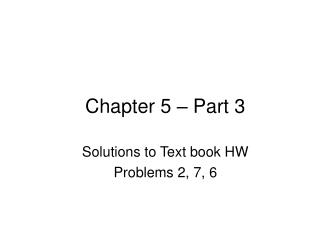DownloadDownload PresentationChapter 5 – Part 3

# Chapter 5 – Part 3

Télécharger la présentation## Chapter 5 – Part 3

- - - - - - - - - - - - - - - - - - - - - - - - - - - E N D - - - - - - - - - - - - - - - - - - - - - - - - - - -
##### Presentation Transcript

1. Chapter 5 – Part 3 Solutions to Text book HW Problems 2, 7, 6

2. Part 1 Part 2 R1 =.90 R2 =.87 Review: Components in Series Both parts needed for system to work. RS = R1 x R2 = (.90) x (.87) =.783

3. R1 =.93 R2 =.90 RBU =.85 Review: (Some) Components in Parallel

4. Review: (Some) Components in Parallel • System has 2 main components plus a BU Component. • First component has a BU which is parallel to it. • For system to work, • Both of the main components must work, or • BU must work if first main component fails and the second main component must work.

5. Review: (Some) Components in Parallel A = Probability that 1st component or its BU works when needed B = Probability that 2nd component works or its BU works when needed = R2 RS = A x B

6. Review: (Some) Components in Parallel A = R1 +[(RBU) x (1 - R1)] = .93 +[(.85) x (1 - .93)] = .9895 B = R2 = .90 Rs = A x B = .9895 x .90 = .8906

7. Problem 2 A jet engine has ten components in series. The average reliability of each component is.998. What is the reliability of the engine?

8. Solution to Problem 2 RS = reliability of the product or system R1 = reliability of the first component R2 = reliability of the second component and so on RS = (R1) (R2) (R3) . . . (Rn)

9. Solution to Problem 2 R1 = R2 = … =R10 = .998 RS = R1 x R2 x … x R10 = (.998) x (.998) x   x (.998) = (.998)10 =.9802

10. RBU =.80 R1 =.90 Problem 7 • An LCD projector in an office has a main light bulb with a reliability of .90 and a backup bulb, the reliability of which is .80.

11. Solution to Problem 7 RS = R1 + [(RBU) x (1 - R1)] 1 - R1 = Probability of needing BU component = Probability that 1st component fails

12. R1 = .90 RS = R1 +[(RBU) x (1 - R1)] RS = .90 +[(.80) x (1 - .90)] = .90 +[(.80) x (.10)] RBU = .80 = .9802 .98 Solution to Problem 7

13. Problem 6 What would the reliability of the bank system above if each of the three components had a backup with a reliability of .80? How would the total reliability be different?

14. R1 = .90 R2 = .89 R3 = .95 RBU = .80 RBU = .80 RBU = .80 Problem 6

15. Solution to Problem 6 – With BU • First BU is in parallel to first component and so on. • Convert to a system in series by finding the probability that each component or its backup works. • Then find the reliability of the system.

16. Solution to Problem 6 – With BU A = Probability that 1st component or its BU works when needed B = Probability that 2nd component or its BU works when needed C = Probability that 3rd component or its BU works when needed RS = A x B x C

17. Solution to Problem 6 – With BU A = R1 +[(RBU) x (1 - R1)] = .90 +[(.80) x (1 - .90)] = .98

18. Solution to Problem 6 – With BU B = R2 +[(RBU) x (1 - R2)] = .89 +[(.80) x (1 - .89)] = .978

19. Solution to Problem 6 – With BU C = R3 +[(RBU) x (1 - R3)] = .95 +[(.80) x (1 - .95)] = .99

20. .98 .978 .99 Solution to Problem 6 – With BU RS = A x B x C = .98 x .978 x .99 =.9489

21. R1 = .90 R2 = .89 R3 = .95 Solution to Problem 6– No BUs RS = R1 x R2 x R3 = (.90) x (.89) x (.95) = .7610

22. Solution to 6 - BU vs. No BU • Reliability of system with backups =.9489 • Reliability of system with backups =.7610 • Reliability of system with backups is 25% greater than reliability of system with no backups: (.9489 - .7610)/.7610 = .25